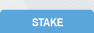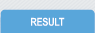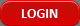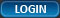HOW TO CALCULATE WINNINGS ?

Winnings on fixed odds are easiest to calculate when the maximum number of lines obtainable have been secured. Just multiply the amount staked by the odd for the coupon that week.

However if in a permutation game, you get at least the minimum selection required, to determine your winnings, first you must know the lines of your selection, then your winning lines which is defined by the numbers you get correctly in your selection. You can then calculate your winning as follows:

(i)                Multiply your winning lines by the amount staked per line;

(ii)             Multiply what you get in (i) above by the odd for the game that week.

For example: If you make a permutation of 3 from 6, the total number of lines is 20. If your total investment on the coupon is N500, that means the coupon was staked at N25 per line. If out of the 6 games, 5 are correct, your winning line is 10. If the odd for the game that week is 40, then your winning will be:

(i)                10 multiplied by 25 = 250

(ii)             250 multiplied by 40 =10,000

However there is a more direct formula for this calculation which is:

Winning          =          WL x CPOD x AS

TLS

Where:

WL      = Winning Lines        = 10

CPD    = Coupon Odd           =  40

AS       = Amount staked       =  500

TLS     = Total Lines staked =  20

Winning          =          10 x 40 x 500             =          10000

20

Steve staked 10 numbers, expecting a minimum of 3, on the Mars GND coupon with N24,000 in week 41. 8 of his selection were correct. How much did he win?

WL      = 56

CPD    = 124

AS       =  24000

TLS     = 120

Winning          =          56 x 124 x 24,000        =          1,388,800

120

That same week, Steve staked 6 numbers expecting a minimum on the Under-4 Away coupon with N1200. 4 of his selections were correct. How much did he win?

WL      = 1

CPD    = 4

AS       = 1200

TLS     = 15

Winning          =          1 x 4 x 1200               =          320

15

But if 5 of his selection had been correct, his winning would have been:

Winning          =          5 x 4 x 1200               =          1,600

15

If Steve had gotten the whole 6 selections correct, his winnings would have been directly calculated as:

Winning          =          CPD x AS

=          4 x 1200                     =          4,800

In the calculation of winnings, the ready reckoner comes very handy in determining the total lines staked and the winning lines.

 Permutation Table: . From 2 3 4 5 6 7 8 9 10 11 12 13 14 15 16 17 18 19 20 2 1 3 6 10 15 21 28 36 45 55 66 78 91 105 120 136 153 171 190 3 1 4 10 20 35 56 84 120 165 220 286 364 455 560 680 816 969 1,140 4 1 5 15 35 70 126 210 330 495 715 1,001 1,365 1,820 2,380 3,060 3,876 4,845 5 1 6 21 56 126 252 462 792 1,287 2,002 3,003 4,368 6,188 8,568 11,628 15,504 6 1 7 28 84 210 462 924 1,716 3,003 5,005 8,008 12,376 18,564 27,132 38,760 8 1 9 45 165 495 1,287 3,003 6,435 12,870 24,310 43,758 75,582 125,970About Us  |  Coupons  |  Results  |  Register  |  Login  |  Contact Us  |  F.A.Q.  |  Stake thru SMS  |  Calculate Winnings © Mars Leisure Limited 2019, All rights reserved. MarsLeisure.com - Privacy Policy Designed & Developed by Portalys